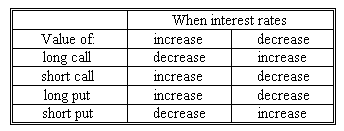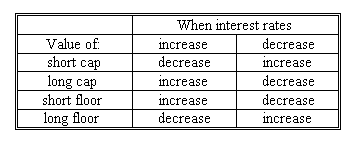### Why should I choose AnalystNotes?

AnalystNotes specializes in helping candidates pass. Period.

### Subject 3. Options on interest rates versus options on fixed income securities

An interest rate cap can be seen as a series of European call options, or "caplets" on a specified reference rate, usually LIBOR. An interest rate floor can be seen as a series of European put options, or "floorlets". The question is what type of package of options is a cap and a floor. It depends on whether the underlying is a rate or a fixed income instrument.

If the underlying is considered a fixed-income instrument, its value changes inversely with interest rates. Therefore:For a cap and floor, the situation is as follows:Therefore, buying a cap (long cap) is equivalent to buying a package of puts (long put) on a fixed-income instrument, and buying a floor (long floor) is equivalent to buying a package of calls (long call) on a fixed-income instrument.

If an option is viewed as one in which the underlying is an interest rate, then buying a cap is equivalent to buying a package of calls on interest rates and buying a floor is equivalent to buying a package of puts on interest rates. For example, for a call option on an interest rate, there is a payoff if the reference rate is greater than the strike rate. When interest rate increases, the call option's value increases. This is a payoff of a long cap.